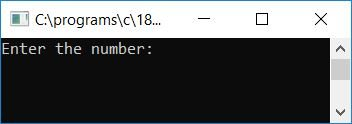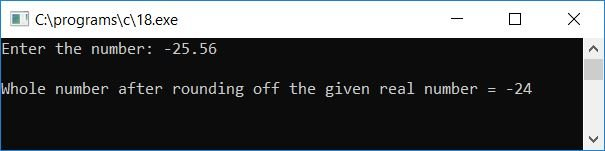# C Program to Round Off a Decimal or Floating-Point Number

In this tutorial, we will learn how to create a program in C that will ask the user to enter any number (any real or floating-point number) to round it off to the nearest integer. For example, if the user has provided 23.43 as input, then the program will output its nearest integer value, which will be 23. Let's take a look at the program:

```#include<stdio.h>
#include<conio.h>
int main()
{
float num;
int tempCheck, roundNum, tempNum;
printf("Enter the number: ");
scanf("%f", &num);
if(num>0)
{
tempNum = num*10;
tempCheck = tempNum%10;
if(tempCheck>=5)
{
roundNum = num;
roundNum++;
}
else
{
roundNum = num;
}
printf("\nWhole number after rounding off the given real number = %d", roundNum);
}
else if(num<0)
{
num = -(num);
tempNum = num*10;
tempCheck = tempNum%10;
if(tempCheck>=5)
{
roundNum = num;
roundNum--;
}
else
{
roundNum = num;
}
printf("\nWhole number after rounding off the given real number = -%d", roundNum);
}
else
{
printf("\nThe given number is 0");
}
getch();
return 0;
}```

Because the above program was written in the Code::Blocks IDE, the output will be similar to the one shown below after a successful build and run. This is the first snapshot of the sample run:Now supply any real number, say 37.5, and press the ENTER key to see the nearest integer (whole number) value of the given real number:Here is another sample run of the above program; this time let's suppose the user has provided the real number, say -25.56, as input:Here is a list of some of the main steps used in the above program:

• Receive any real number as input.
• Now Use two if statements to round it off. First, when the user has supplied the real number, which is positive. Second, when the user has supplied the real number, which is negative.
• Now if the given number is a positive number, then multiply the number by 10 and initialize it to a variable, say tempNum. Then find the remainder of tempNum (by dividing by 10) and initialize it to another variable, say tempCheck. Check whether the tempCheck variable holds a value that is greater than 5 or not. If it is, then initialize the value of num to another variable, say roundNum, and increment it by 1. Then, at last, print the value of roundNum, which will be the nearest integer value of the given number.
• And if the given number is a negative number, then first make it a positive number just by multiplying the number with the minus (-) operator. Do the same steps as done in the case of a positive number, except that this time we have to decrement the value of roundNum, as in the case of a negative number, a greater number means a smaller value. That is, 34 is greater than 33, but -34 is less than -33.
• For example, if the user has supplied 33.45 as input, then program flow goes inside the first if block because 33.45 is greater than 0.
• Inside the if block, num*10 or 33.45*10 or 334.5 will be initialized to tempNum, and tempNum%10 or 334.5%10 or 4 will be initialized to tempCheck.
• Note: Here we have multiplied the number by 10 and then applied the modulus operator (%) to make the first digit present after the decimal come before the decimal. So that we can check the number by using the modulus operator to see if it is greater than or equal to 5 (that comes after the decimal). That is, if the number is greater than or equal to 5, then we have to increment the value and print it as the nearest integer of the given number, and if the number is less than 5, then print the number as it is (without having a decimal part).
• Now come to the example. As tempCheck holds 4, which is less than 5, the program flow goes inside the else block, and num will be initialized to roundNum. And print the value of roundNum.
• And if user has provided -34.67 as input, then, as the number is less than 0, the program flow goes inside the else if block.
• The number is multiplied by the minus operator (-) and initialized to the number itself, for example, -(num) or -(-34.67) or 34.67 will be initialized to num, num*10 or 34.67*10 or 346.7 will be initialized to tempNum, and tempNum%10 or 346.7%10 or 6 will be initialized to tempCheck.
• As the tempCheck variable holds a value that is greater than 5, program flow goes inside the if block, num will be initialized to roundNum, and the value gets decremented. Finally, print the value, which will be 33, as output.

C Quiz

« Previous Program Next Program »